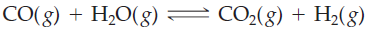×
Get Full Access to Introductory Chemistry - 5 Edition - Chapter 15 - Problem 54p
Get Full Access to Introductory Chemistry - 5 Edition - Chapter 15 - Problem 54p

×

# Consider the reaction. An equilibrium mixture of this reaction at a certain temperatureISBN: 9780321910295 34

## Solution for problem 54P Chapter 15

Introductory Chemistry | 5th Edition

• Textbook Solutions
• 2901 Step-by-step solutions solved by professors and subject experts
• Get 24/7 help from StudySoup virtual teaching assistantsIntroductory Chemistry | 5th Edition

4 5 1 319 Reviews
24
3
Problem 54P

Consider the reaction.An equilibrium mixture of this reaction at a certain temperature has [CO] = 0.0233 M, [H2O] = 0.0115 M, [CO2] = 0.175 M, and [H2] = 0.0274 M. What is the value of the equilibrium constant at this temperature?

Step-by-Step Solution:
Step 1 of 3

Solution: Here, we are going to find the value of the equilibrium constant for the given reaction at the given temperature.

Step1:

The equilibrium constant for a general reaction,

aA + bB ⇄ cC + dD

is expressed as,

Kc = [C]c [D]d / [A]a [B]b

Where [A], [B], [C] and [D] are the equilibrium concentrations of the reactants and products.

Step2:

For the reaction,         CO(g) + H2O(g) ⇄ CO2(g) + H2(g)

Kc = [CO2] [H2] / [CO] [H2O]

Given,

[CO2] = 0.175 M

[CO] = 0.0233 M

[H2] = 0.0274 M

[H2O] = 0.0115 M

Substituting the values in the above equation, we get,

Kc = (0.175 x 0.0274) / (0.0233 x 0.0115]

= 0.004795 / 0.00026795

= 17.895

Thus, the value of the equilibrium constant is 17.895.

------------------------------

Step 2 of 3

Step 3 of 3

##### ISBN: 9780321910295

This full solution covers the following key subjects: temperature, reaction, Equilibrium, mixture, constant. This expansive textbook survival guide covers 19 chapters, and 2046 solutions. The answer to “?Consider the reaction. An equilibrium mixture of this reaction at a certain temperature has [CO] = 0.0233 M, [H2O] = 0.0115 M, [CO2] = 0.175 M, and [H2] = 0.0274 M. What is the value of the equilibrium constant at this temperature?” is broken down into a number of easy to follow steps, and 42 words. Introductory Chemistry was written by and is associated to the ISBN: 9780321910295. Since the solution to 54P from 15 chapter was answered, more than 299 students have viewed the full step-by-step answer. The full step-by-step solution to problem: 54P from chapter: 15 was answered by , our top Chemistry solution expert on 05/06/17, 06:45PM. This textbook survival guide was created for the textbook: Introductory Chemistry, edition: 5.

Unlock Textbook Solution